# PSEB 10th Class Science Important Questions Chapter 10 Light Reflection and Refraction

Punjab State Board PSEB 10th Class Science Important Questions Chapter 10 Light Reflection and Refraction Important Questions and Answers.

## PSEB 10th Class Science Important Questions Chapter 10 Light Reflection and Refraction

Question 1.
(a) Form the image in case an object is moved from infinity to the concave mirror.
(b) Describe with the help of diagram the nature, size and position of the image formed when an object placed at centre of curvature of a concave mirror.
(a) Images formed by concave mirror when it is moved from infinity to the mirror
1. Object is at Infinity: Two cases arise :

• When mirror is in parallel plane to the object: In such a case, rays from infinity come parallel to principal axis. After reflection they pass through principal focus F. Image is extremely small, real, inverted and it is formed at the principal focus [Figure (a)].
• When the rays strike the mirror obliquely. The ray AB passing through F after reflection goes parallel to principal axis towards BA’. Another ray DE through C striking normally to the mirror at E is reflected back. The two rays form an image at A’. Image is real, inverted, extremely diminished and is formed at F [Figure (b)].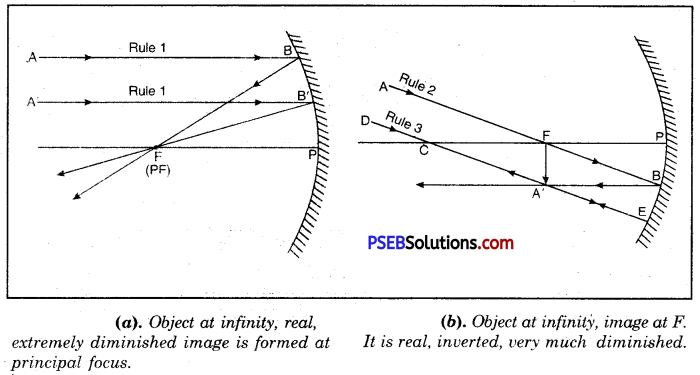2. Object beyond C. A ray of light AD from A parallel to principal axis after reflection passes through F.
Another ray from A passes through C, and is reflected back along the same path, forming real, diminished, inverted image A’B’, between F and C. [Figure (c)].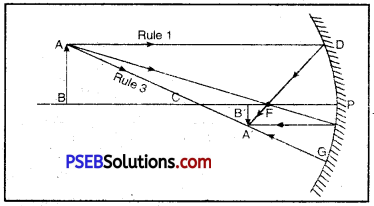(c) Object beyoid C. a real, inverted, diminished image hetiveen F and C. inverted at C and is of saine size as that of object.

3. Object at centre of curvature, C i.e., at 2f. A ray AD from A parallel to principal axis after reflection from mirror passes through F.
Another ray AD’ from A through F. goes, parallel to principal axis i.e., towards, D A forming real, inverted image of AB at C i.e., at 2f. The image is of the same size as the object [Figure (d)].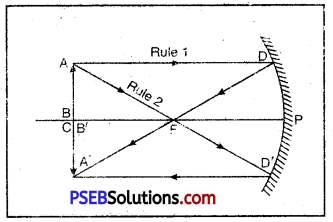(d) Object at C. Image is also at C. It is real.

4. Object between F and C between [f and 2f]
A ray AD from object going parallel to principal axis is reflected towards F. Another ray AE as of coming through C is reflected back forming image of A at A’. Similarly image of B is formed at B’. Image is real, inverted, enlarged and formed beyond C (2f) i.e., as shown in Figure (e).(e) Object between F and C. Image is beyond C. It is real, inverted and enlarged.

5. Object at F. A ray AD parallel to principal axis passes through F. Another ray AE strikes the mirror normally at E is reflected back as it appears to pass through C. This reflected ray becomes parallel to reflected ray DY and, therefore, forms an image of object AB at infinity. The image is very much enlarged and is real and inverted [Figure (f)].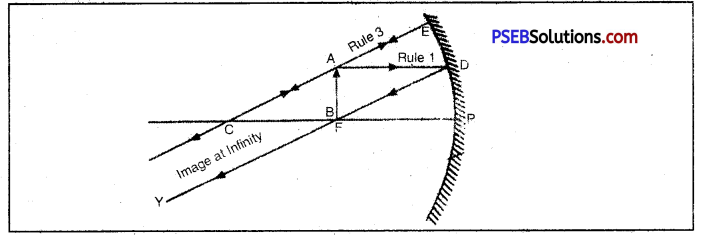(f). Object at F. Real, inverted, extremely enlarged image is formed at infinity.

6. Object between F and P (Principal Focus and Pole).
A ray AD from A goes parallel to principal axis after reflection passes through F. Another ray AE striking the mirror normally through C is reflected back. These to reflected rays do not actually meet but meet when produced backward, therefore, they form virtual image of the object behind the mirror. The image is erect and enlarged and formed behind the mirror [Figure (g)].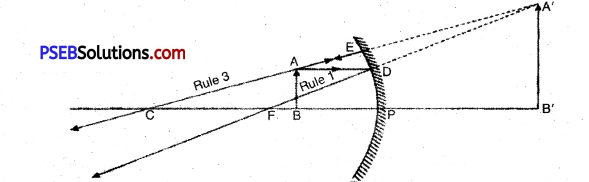(g). Object between F and P. An erect, enlarged, virtual image is formed behind the mirror.
(b) Please see Figure(d) and its description given on previous page.

Question 2.
What is refraction of light? with the help of diagram explain refraction of light by rectangular glass slab and show that emergent ray and incident ray are parallel.
Refraction of Light. When a ray of light enters from one medium to another medium then it changes its path at the meeting plane of two media. This phenomenon of light is called refraction of light.

Refraction by glass slab.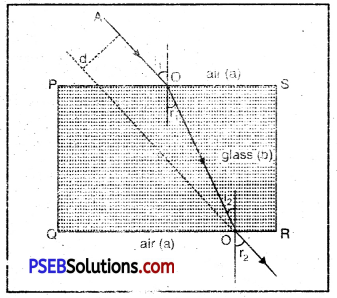Refraction by rectangular glass slab

A rectangular glass slab PQRS is placed in air. When? ray of ligxu, in air enters glass slab then AO, OO’ and O’B are incident ray, refracted ray and emergent ray respectively

By applying snell’s Law at ‘O’ $$\frac{\sin i_{1}}{\sin i_{1}}$$ = aµb ….(i)

Now ray of hgnt is uavelling from glass (denser medium) to air (rarer medium).
Again using sneirs Law at O
bµa = $$\frac{\sin i_{2}}{\sin r_{2}}=\frac{\sin r_{1}}{\sin r_{2}}$$ ……….(iii) [∵∠i2 = ∠r1]

According to principle of reversibility of light
bµa = $$\frac{1}{{ }^{a} \mu_{b}}$$

From (ii) and (iii)
aµb = $$\frac{\sin r_{2}}{\sin r_{1}}$$ ……..(iv)
Comparing (i) and (iv)
$$\frac{\sin i_{1}}{\sin r_{1}}=\frac{\sin r_{2}}{\sin r_{1}}$$
or sin i1 = sin r2
∴ ∠r1 = ∠r2
This means that angle of incidence is equal to emergent angle.

Therefore, when refraction of light takes place in a rectangular glass slab then emergent ray and incident ray are equal.Question 3.
Draw the ray diagrams and find position, nature and size of image formed by a convex lens, when object is placed:
(i) Between F and 2F
Object between F and 2F. When the object is between F and 2F, its real. inverted, magnified image is formed on the other side of the convex lens as shown in Figure (a).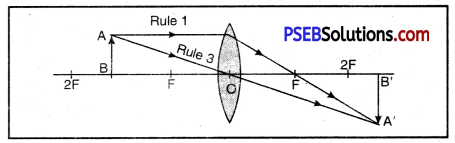(a) Object bet weer F and 2F real, inverted, magnified image is formed beyond 2F on the other side of lens.

(ii) Beyond 2F
Object beyond 2F’. When the object is beyond 2F, a real, inverted, diminished image is formed between F’ and 2F [Fig (b)].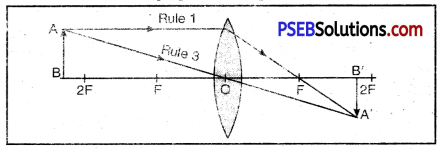(b) Object beyond 2F, real, inverted, diminished image between Fund 2F

(iii) At F.
Object at F. When object is placed at principal focus, a real, inverted and highly magnified image is formed at infinity [Fig. (c).]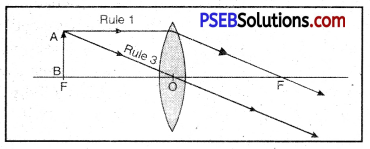(c) Object at F, highly magnified, real, inverted image is formed at infinity.

Question 4.
Give the nature, position and size of the image formed by a convex lens when the object lies at 2F and between focus F and optical centre O.
1. Object at 2F. A ray AD from point A of the object going parallel to principal axis, passes through F on the other side of the lens goes towards DA’. Another ray from A through optical centre O goes towards OA’. The two meet at A’ forming image of A at A’. Image of object AB is formed at 2F on the other side of the lens. Image is real, inverted and of the same size as that of object [Figure (a)].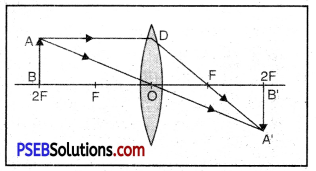2. Object between F and O. When an object is placed between principal focus and optical centre of the lens, virtual, erect, magnified image is formed on the same side of the lens [Figure.(b)].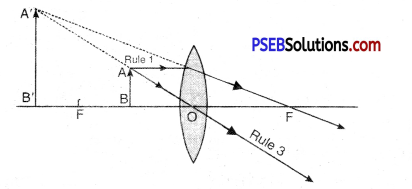(b) Object between F and O, a virtual. erect, magnified image is formed on the same side.

Question 1.
What is light? Give the nature of light.
Light. Light is a form of energy which produces in us the sensation of sight. Light itself is not seen but it helps us to see the surrounding objects.

Nature of light. Light has a dual nature, a wave nature and a particle nature. To explain the phenomenon it was thought of as a wave but when it could not explain the treatment of interaction of light with matter then light was believed to have particle nature. The confusion about true nature of light was later explained when Model Quantum Theory emerged according to which “light is neither a wave nor a particle”. Modern Quantum Theory reconciles the particle properties of light with the wave nature

i. e. light is emitted as a particle but travels in the form of wave.

Question 2.
Write the characteristics of light.

• It does not require medium for its propagation.
• It is in the form of electro-magnetic waves.
• Its speed depends upon the nature of medium through which it passes.

Question 3.
What are the various artificial sources of light? Give examples.
Artificial Sources of Light: The main artificial sources of light are : fire, electricity gas and some chemical reactions.

Question 4.
What is Reflector?
Reflector. Any smooth and polished surface that returns light into the same medium from which it is coming, is called reflector.

Question 5.
What is reflection of light? State the laws of reflection of light.
Reflection of Light. When rays of light fall on a smooth and polished surface (incident) then they come back into the same medium in a particular direction. This phenomenon of coming back of light is known as reflection of light.

Laws of Reflection. There are two laws of reflection :

• The angle of incidence is equal to the angle of reflection i.e. ∠i = ∠r.
• The incident ray, the reflected ray and the normal at the point of incidence all lie in the same plane.
• In fig. AB is a reflecting surface (plane mirror), PQ is incident ray. QR is reflected ray and QN is normal at the point of incidence Q.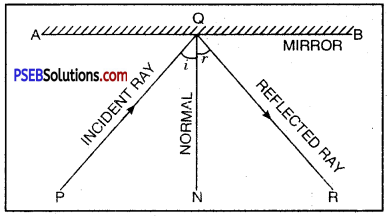Figure shows that incident ray, reflected ray and the normal all lie in the same plane of paper.

Question 6.
What is the angle of incidence when incident ray falls normal to the mirror?
When a ray of light is incident normal to the mirror then the angle of incidence is zero (i.e. ∠i= 0°).

Question 7.
What is the angle of reflection when a ray of light is incident normally to the mirror?
When a ray of light is incident to the mirror normally (∠i = 0°) then after reflection it returns along the same path. In that case, angle of reflection will also be zero (∠r = 0°).

Question 8.
A ray of light falling normal to the mirror returns along the same path. Why?
A ray of light falling normal to any mirror returns along the same path. In that case ∠i = 0° and according to the law of reflection ∠i = ∠r. So ∠r will be equal to zero and the reflected ray would be along the same path.

Question 9.
Define the terms :
(i) Spherical mirror
Spherical Mirror: If the mirror is a part of some hollow sphere whose one surface is polished and the other surface is reflecting then such a mirror is called spherical mirror.

Spherical mirrors are of two types :
(a) Concave mirror
(b) Convex mirror.

(ii)Concave mirror
Concave Mirror: A spherical mirror whose reflecting surface is towards the centre of that sphere of which the mirror is a part is called concave mirror. The outer surface of concave is polished and reflection occurs from the inner surface.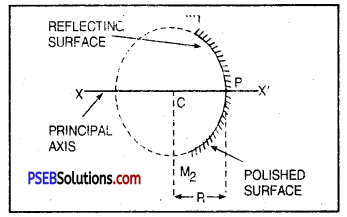Concave mirror

(iii) Convex mirror
Convex Mirror: A spherical mirror whose reflecting surface is away from the centre of that sphere of which the mirror is a part, is called convex mirror.
The inner surface of convex mirror is polished and reflection takes place from the outer surface.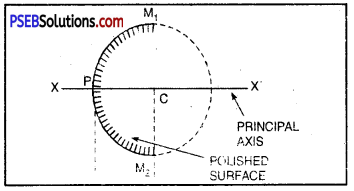Convex Mirror

(iv) Aperture.
Aperture: That part of the mirror from which reflection takes place is called aperture of the mirror.
In figure (a) and (b) the length M1M2 is aperture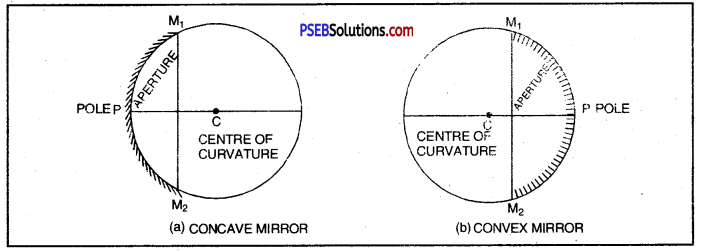Aperture and Centre of Curvature

Question 10.
Define the terms :
(i) Centre of Curvature
Centre of Curvature: It is the centre of that hollow sphere of which the mirror is a part. In the above figure (a) C is a centre of curvature of concave mirror and in figure (b) C is centre of curvature of convex mirror.

(ii) Pole
Pole. The mid point of spherical mirror is called its pole or vertex. In the fig.l0.14.(a) and fig.10.14.(6) given below P is pole.

(iii) Principal Focus
Principal Focus: The principal focus of the mirror is a point on the principal axis where parallel rays of light after reflection from the mirror actually meet at a point or appear to diverge.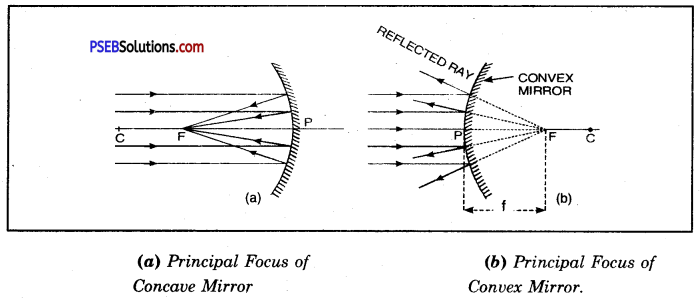(iv) Focal length.
Focal Length: The distance between pole and focus is called focal length of the mirror. It is denoted by ‘f. In fig. 10.14. PF is focal length. In SI system the unit of focal length is metre (m).Question 11.
What is the relation between focal length and radius of curvature of a concave mirror? What is focal length of a plane mirror?
The focal length of a concave mirror is half the radius of curvature of that mirror. If ‘f is the focal length and ‘R‘ the radius of curvature of concave mirror, then
f = $$\frac{1}{2}$$ × R
The focal length of plane mirror is infinite (∞).

Question 12.
When the image formed by a concave mirror is at infinity then what is the position of the object?
When the object is placed at focus of a concave mirror then the image of the object is formed at infinity. This image is real, big in size and is bigger then the size of the object. In this position the rays of light coming from the object after suffering reflection from the mirror become parallel.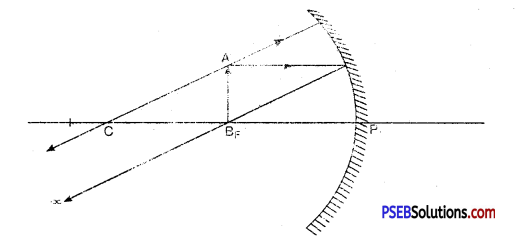Reflected rays meet at Infinity.

Question 13.
Where should an object be placed so that its real and same size image is formed?
To get real image of saine size being formed by a concave mirror, the object should be place at its centre of curvature. In this case the image will also be formed at the same position i.e at centre of curvature. This image will be real, inverted and of the same size of the object.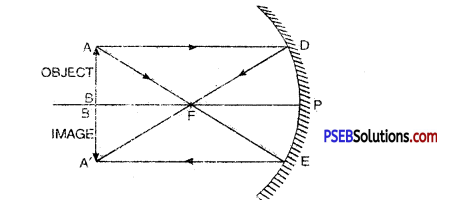Question 14.
When is virtual and magnified image of an object formed in a concave mirror. Show with the help of a diagram.
When an object is placed between pole and focus of a concave mirror then in that case a virtual, erect and magnified image is formed with respect to the size of the object.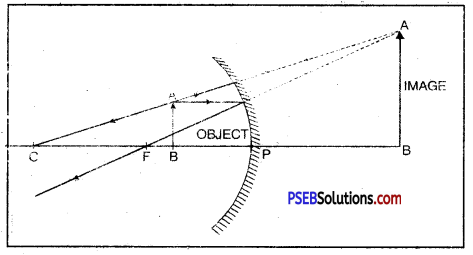Question 15.
Which mirror is shown in the figure? Where is object placed with respect to the mirror? Write one characteristic of the image formed.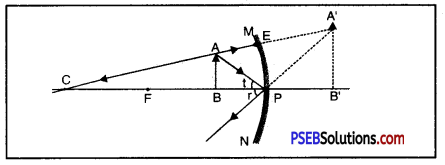(a) Concave Mirror.
(b) Object is placed between Focus and Pole of the mirror.
(c) Characteristic of Image formed. The image is virtual, erect and magnified and is formed behind the mirror.

Question 16.
Which mirror is shown in the diagram? Where is object placed in relation to the mirror? Write characteristics of the image formed :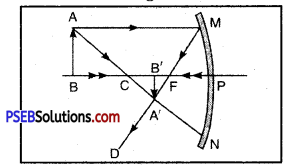In the diagram concave mirror is shown.
The object AB is placed beyond centre of curvature of the mirror i.e. it is at a distance more than radius of curvature (r = CP) from the pole of the mirror.

Characteristics of image formed
The image ¡VB’ of the object AB is :

• Real and inverted
• The image is smaller in size as compared to the size of the object.

Question 17.
Which mirror is used as a shaving mirror and why? Explain its working with the help of a ray diagram.
Concave mirror is used as a shaving mirror because we place our face close to the mirror (in between Pole and Focus), the image formed is erect and magnified. This makes the fine hairs to appear quite big so that we can shave properly.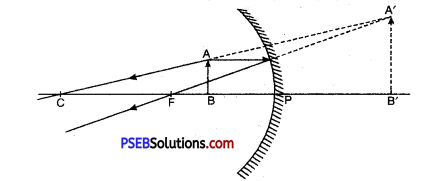Question 18.
Which mirror always forms virtual, erect and smaller image?
Convex mirror always forms a virtual, erect and reduced image of whatever may be the position of the object. The image is formed behind the mirror as is shown in figure.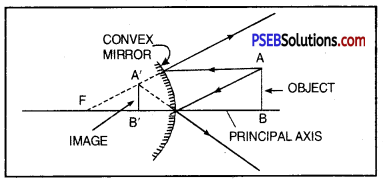Formation of Image in a Convex Mirror

Question 19.
Which mirror has wider filed of view?
In a convex mirror the image formed is always virtual and smaller in size as compared to the size of the object. This image is formed behind the mirror. On moving the mirror away, the images of objects lying over the large area can be seen in the mirror. Therefore, its area of view becomes wide.

Question 20.
Which mirror is preferred as a driver’s mirror and why? Draw a ray diagram.
Convex mirror is preferred as a driver’s mirror because the image formed by convex mirror is erect and smaller in size. Therefore, it enables the driver to see traffic coming behind over large area.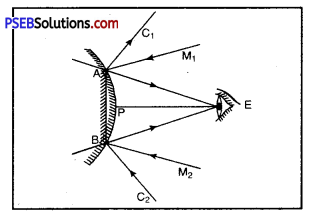A convex mirror as a driver’s mirror

Question 21.
Where should an object be placed with respect to a concave mirror to get real and enlarged image? Show with the help of ray diagram.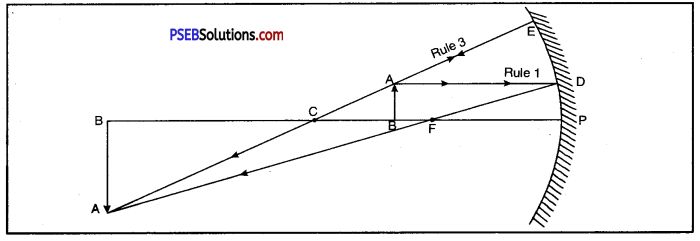Object between F and C. Image ¿s beyond C. It is real, inverted and enlarged.

Question 22.
Write points of difference between convex mirror and concave mirror.
Differences between Convex Mirror and Concave Mirror.

 Convex Mirror Concave Mirror 1. In convex mirror the shining reflecting surface is bulged outward. 1. In concave mirror shining reflecting surface is depressed inward. 2. Convex mirror forms virtual image. 2. In concave both real and virtual images are formed. 3. In convex mirror the image formed is erect. 3. In concave mirror both erect and inverted images are formed. 4. In convex mirror the image formed is always smaller in size whatever may be the position of the object. 4. In concave mirror all types of images : bigger, smaller or of the same size are formed depending upon the position of the object.Question 23.
How will you distinguish between plane mirror, convex and concave mirror without touching?
Look through all these mirrors turn by turn.

• In one of the mirrors, we shall observe that the image is erect, of the same size as the object and as far behind the mirror as the object is in front of it. It is plane mirror.
• In another mirror, the image is always diminished and erect. This is convex mirror.
• In third mirror, image will appear erect and very large when seen by placing eyes, close to the mirror. However, when the eyes are placed at a distance, image will be always inverted. This mirror is concave.

Question 24.
Define magnification of spherical mirror. What is the magnification produced in a plane mirror?
Or
What is magnification?
Magnification: Magnification of spherical mirror is defined as the ratio of the size of the image formed by the mirror to the size of the object. It is denoted by ‘m’.
∴ Magnification (m) = $$\frac{\text { Size of the image (I) }\left(h_{2}\right)}{\text { Size of the object (O) ( } h_{1} \text { ) }}$$
m = $$\frac{\mathrm{A}^{\prime} \mathrm{B}^{\prime}}{\mathrm{AB}}=\frac{-v}{u}$$

Magnification in a Plane Mirror. Plane mirror can be considered to be a part of such a sphere whose radius of curvature is infinity.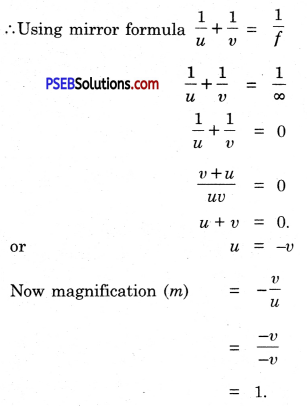Hence, the size of the object and the size of the image formed in a plane mirror are equal in size.

Question 25.
Write the characteristics of image formed in a plane mirror.
Characteristics (or properties) of image in a Plane Mirror.

• It is virtual i.e. this image can not’ be obtained on a screen.
• It is erect.
• It is laterally inverted i.e. the right hand of the object appears left hand of the image and the left hand becomes the right hand.
• The size of the image formed in a plane mirror is equal to the size of the object.
• The image formed in a plane mirror is as far behind as the object is in front of it.

Question 26.
Write difference between real image and virtual image formed by a mirror.
Differences between Real Image and Virtual Image.

 Real Image Virtual Image 1. It is formed by the actual intersection of reflected rays. 1. It is formed by the virtual intersection of reflected rays. 2. It is inverted. 2. It is erect. 3. It can be obtained on the screen. 3. It can not be obtained on the screen.

Question 27.
Explain with the help of a diagram the formation of image formed by a plane mirror.
Or
How will you show that the image formed in a plane mirror is as far behind the plane mirror as the object is in front of it?
Image formed by a Plane Mirror. Suppose MM’ is a plane mirror and 0 is a point object lying in front of it. From ‘O’ consider a ray of light ON falling normally to the mirror which retraces its path. Now consider two rays of light OA and OB starting from the object which are incident to the plane mirror. These rays after reflection from the plane mirror form two reflected rays AC and BD. These two reflected rays do not meet but appear to meet at ‘I’ where the image is formed. This ‘I’ is the virtual image of the object ‘O’.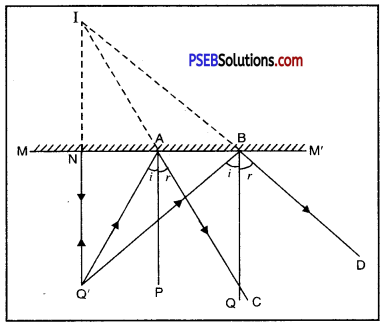On measurement, we find that NO = NI
i. e. Distance of the object from the mirror = Distance of the image from the mirror.
Therefore, the image formed in a plane mirror is as far behind the mirror as the object is in front of it.

Question 28.
Write uses of spherical mirrors.
Or
What are the uses of concave and convex mirrors?
Uses of Spherical Mirrors. Spherical mirrors are of two types

1. Concave mirror
2. Convex mirror.

1. Uses of Concave Mirror:

• Large sized concave mirrors are used in solar cookers to focus solar energy on an object to be heated. Due to a limited reserve of oil and gas, the importance of solar cookers can no longer be ignored.
• Concave mirrors are used as reflectors in car head-lights, search-lights, torches and table lamps.
• Concave mirrors can be used as a shaving mirror or make up mirror. When the concave mirror is placed at a distance less than its focal length from the face, a magnified erect image is produced. Hence any cut or left over hair or minor flaw in the make up can easily be observed with the help of a concave mirror.
• Concave mirrors are used by E.N.T. doctors to concentrate hght on ear, nose, throat, etc.

2. Uses of Convex Mirror:

• Convex mirrors are used in automobiles (e.g., scooters, cars, trucks, buses, etc.) to see bulk of rear traffic. The advantage of using a convex mirror is that it gives a very larger field of view of the rear traffic than a plane mirror.
• The disadvantage of convex mirror is that since the image is always formed between P and F, we cannot accurately judge the distance and speed of the vehicles approaching from behind.
• Usually two mirrors, one plane and the other convex are used to see rear traffic whereas convex mirror on side of vehicle gives wider field of view, plane mirror in front of driver helps us in judging the distance and speed of the vehicles coming behind.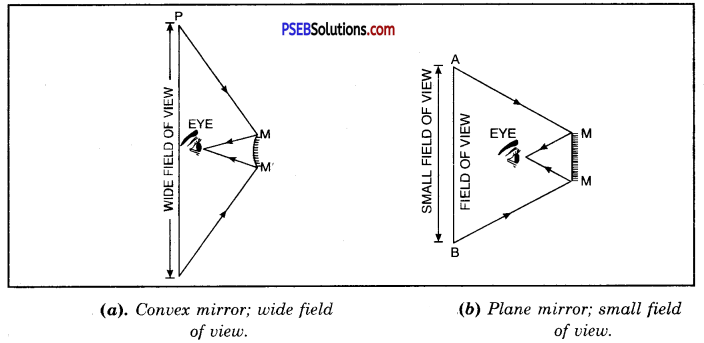Question 29.
What are New Cartesian Sign Conventions used for reflection in spherical mirror? What is the mirror formula?
New Cartesian Sign Conventions for Spherical Mirrors.
The modern cartesian sign conventions are :

• All the rays are drawn from left to right.
• All distances are measured from the pole of the spherical mirror.
• The distances measured in the direction of the incident light are taken as positive and those measured in a direction opposite to the direction of incident light are taken as negative.
• The heights measured upwards and perpendicular to the principal axis of the mirror are taken as positive and vice versa.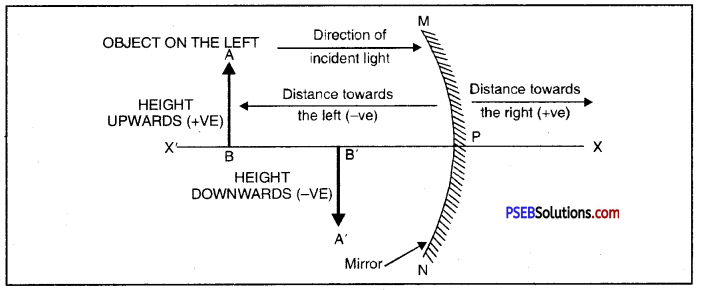Since the object is always placed on the left of the mirror therfore, u is always negative.

Question 30.
What is the effect of density on ray of light when light enters from a rarer medium to a denser medium? Explain with diagram.
Or
What is the effect of density on bending of refracted ray during refraction?
When a ray of light travelling from rarer medium enters into a denser medium then it bends towards the normal. The more the medium is denser, the more it will bend towards the normal. In the figures given below ray of light (a) from air to water (b) air to glass is shown. The bending of light is more in glass than in water because glass is more dense with respect to water.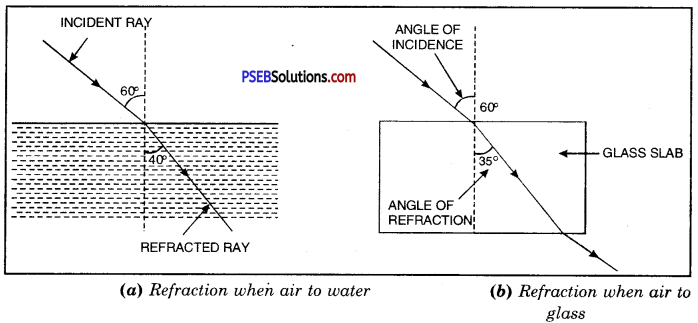Question 31.
When light enters from water to glass what is the change in its velocity?
Water is a rarer medium with respect to glass. Therefore, when light enters from water to glass then velocity of light decreases. It suffers refraction and bends towards the normal. In this case angle of incidence (∠i) is greater than the angle of refraction (∠r).

Question 32.
If a ray of light travelling in glass enters into water will it bend towards or away from the normal?
When light travels from glass medium (denser medium) enters into water (rarer medium), it suffers refraction and bends away from the normal. In this case angle of incidence (∠i) will be smaller than the angle of refraction (∠r) i.e. ∠i < ∠r and also the velocity of light in water will increase.

Question 33.
Find relation for refractive index in terms of real depth and apparent depth.
Suppose an object is lying in the position A at the bottom of water tank. A ray of light AB starting from A strikes the surface of water normally and after refraction goes along BD. Another ray AC makes angle i with the normal at C and makes an angle of refraction r and reaches our eye. In this way A appears at A’. If g is the refractive index of water then
aμw = $$\frac{1}{{w} \mu_{a}}$$ = μ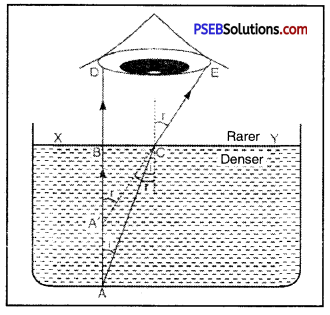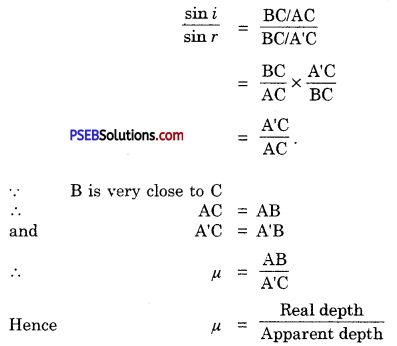Question 34.
Why does a pencil immersed in water appear bent and short? Explain with the help of a ray diagram.
A pencil half immersed in water appears to be bent and short due to refraction of light.
Consider a pencil AB such that its portion CB is immersed in water as shown in figure.

Consider rays of light starting from point B, the tip of the pencil. These rays travel from water (denser medium) and as they enter into air (rarer medium) bend away from normal. The refracted rays appear to come from point B’ slightly raised above. Thus, the immersed portion CB of the pencil appears bent at the surface of water as CB’.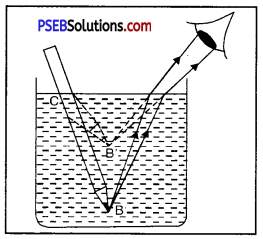Question 35.
Which phenomenon is shown in the figure? Give its definition and give laws of this phenomenon.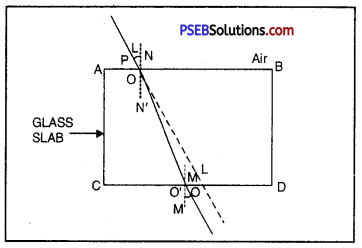The phenomenon of refraction of light is shown in the figure.

Refraction of light: When a ray of light travelling in one transparent medium enters into another transparent medium, it bends at the line of separation of two media. This change of path of hght in second medium is called refraction of light.

Laws of refraction are :

• Incident ray, normal and refracted rays all lie in the same plane.
• Ratio of sine of angle of incidence to the sine of the angle of refraction is constant
i.e., $$\frac{\sin i}{\sin r}$$ = µ, where µ is a constant called refractive index of second medium with respect to first medium .

Question 36.
Define Snell’s Law.
Snell’s Law: The second law of refraction is known as Snell’s law. According to this law, ratio of sine of angle of incidence (sin i) to the sine of angle of refraction (sin r) is constant.

$$\frac{\sin i}{\sin r}$$ = constant.

Question 37.
What is refractive index? Write its mathematical formula.
Refractive Index: The ratio of velocity of light in air or vacuum to the velocity in a medium is called refractive index of medium.
∴ aµb = $$\frac{\text { velocity of light in vacuum }}{\text { velocity of light in medium }}$$
Refractive index has no unit. Since it is ratio of two similar quantities, therefore, it is expressed in number.

Question 38.
What is lens?
Lens: A lens is a piece of transparent and refracting medium bounded by two surfaces either having both the surfaces spherical or one spherical and the other plane surface.

Types of lens. There are two main types of lens :

1. Convex lens (also called converging lens)
2. Concave lens (also called diverging lens)

Question 39.
Define the terms :
1. Optical centre
Optical Centre: The mid point of lens is called optical centre of lens.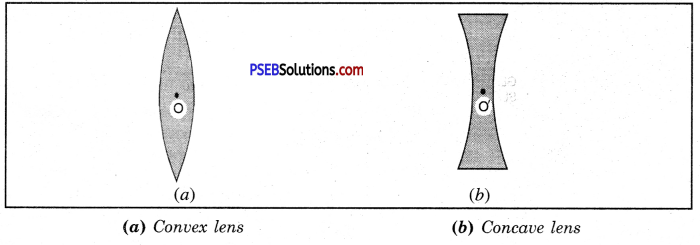In figure (a) O is optical centre of convex lens and in figure (b) O’ is optical centre of concave lens. Any ray of light passing through this point goes undeviated.

2. Principal axis
Principal Axis: The principal axis of a lens is an imaginary line which passes through its optical centre and is normal to the spherical surface of the lens. In figure (a) and figure (b) EF is principal axis of convex and concave lens respectively.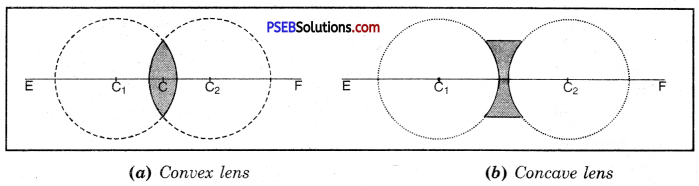3. Principal focus of lens.
Principal Focus of Lens: It is a point on the principal axis where, rays of light coming parallel to the principal axis after refraction meet in case of convex lens and appear to meet on producing backward in case of concave lens.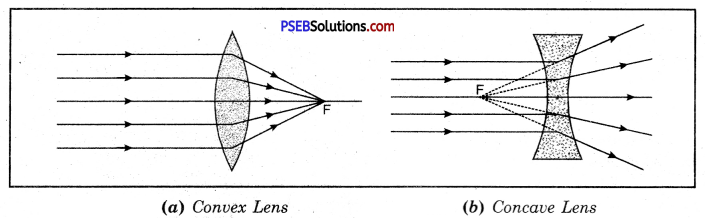Question 40.
How is image formed by a concave lens ? Show by drawing diagram as to what will be the position and nature of the image formed by a concave lens?
The ray diagram for image of an object placed in front of concave lens is shown in figure. The image formed by concave lens always lies between its optical centre and focus. This image is virtual, erect and small in size.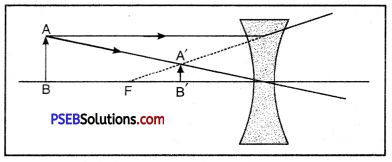Question 41.
What is lens formula? Give its sign conventions and assumptions.
Lens formula. It is the relationship between the distance of object (u), the distance of image (v) from optical centre of the lens, and focal length (f).
i.e. $$\frac{1}{f}=\frac{1}{v}-\frac{1}{u}$$

The formula is equally applicable to convex, concave or any other type of lens (e.g., concavo-convex, plano-convex, convexo-concave, plano-concave etc.)

Sign Conventions.

• The object is always placed to the left of the lens.
• All distances parallel to principal axis are measured from optical centre of the lens.
• All distances measured to the right of optical centre are taken as positive while those measured to the left of the optical centre are taken as negative.
• Distances measured perpendicular to and above the principal axis are taken as positive.
• Distances measured perpendicular to and below the principal axis are taken as negative.

Assumptions.

• Object is taken on the principal axis.
• The lens is thin.

Question 42.
What is magnification of a lens?

• Magnification of a lens is defined as the ratio of the height of the image formed by the lens to the actual height of object.
• If h is the height of the object and h’, the height of the image formed by lens, then magnification,
m = $$\frac{h^{\prime}}{h}$$
• If u and v are the distances of object and image respectively from the optical centre, then
m = $$\frac{h^{\prime}}{h}=-\frac{v}{u}$$

Question 43.
Compare convex and concave lenses.
Differences between Convex Lens and Concave Lens.

 Convex Lens Concave lens 1. Convex lens is thick at centre and thin on the edges. 1. Concave lens is thick on edges and thin at centre. 2. Image is magnified when lens is placed at a distance of less than focal length. 2. Image formed is always diminished. 3. It converges the rays of light. It brings the rays nearer. 3. It diverges the rays i.e., take the rays away from each other.

Question 44.
What are the differences between reflection and refraction?
Differences between Reflection and Refraction.

 Reflection Refraction 1. The incident rays are sent back in the same medium. 1. After refraction, rays pass from one medium to other medium. 2. Angle of incident and that of reflection are equal. 2. Angle of refraction is not equal to angle of incident. Angle r is less or more than angle i depending upon the two mediums.Question 45.
Define 1 dioptre of power of a lens.
Power of a lens is reciprocal of focal length.
P = $$\frac{1}{f}$$

If f = 1 m, P = $$\frac{1}{f}=\frac{1}{1}$$ =1 dioptre
Dioptre: Dioptre or 1 dioptre is the power of a lens of focal length 1 metre.

Numerical Problems

Question 1.
The radius of curvature of a concave mirror is 30 cm. What is its focal length?
Here, Radius of curvature of concave mirror (R) = – 30 cm
Focal length of the mirror (f) =?
We know that R = 2f
or f = $$\frac{R}{2}$$
= $$\frac{-30}{2}$$
∴ Focal length of concave mirror (f) = – 15 cm

Question 2.
The radius of curvature of a convex mirror is 40 cm. What is its focal length?
Here, Radius of curvature of convex mirror (R) = + 40 cm
Focal length of convex mirror (f) = ?
We know that R = 2f
or f = $$\frac{R}{2}$$
= $$\frac{+40}{2}$$
∴ Focal length of convex mirror (f) – + 20 cm

Question 3.
A concave mirror produces three times magnified real image of an object placed at 10 cm in front of it. Find where will the image be formed?
Here object distance (u) = – 10 cm
Magnification (m) = $$-\frac{v}{u}$$ [Real image]
Image distance (v) = ?
We know m = $$-\frac{v}{u}$$
– 3 = $$-\frac{v}{-10}$$
– 3 = $$\frac{v}{10}$$
v = – 3 × 10
= – 30 cm
Negative sign shows that the image is formed at a distance of 30 cm in front of a concave mirror.

Question 4.
An object is placed at a distance of 10 cm from a convex mirror of focal length 15 cm. Find the position and nature of the image.
Sol. Here, focal length of convex mirror if) = + 15 cm
object distance from convex mirror (u)= – 10 cm
Image distance (v) =?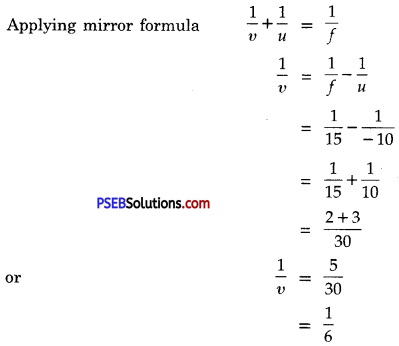∴ Image distance v = + 6 cm
Positive sign shows that the image is formed behind the mirror (on the other side of the mirror) at a distance of 6 cm. This image is virtual and erect.Question 5.
Light enters from air to diamond having refractive index 2.4. What is the speed of light in diamond? Given speed of light in vacuum = 3 × 108 m s-1.
Speed of light in Vacuum, c = 3 × 108 m s-1 Refractive index of diamond, n = 2.4
Speed of light in diamond, vd =?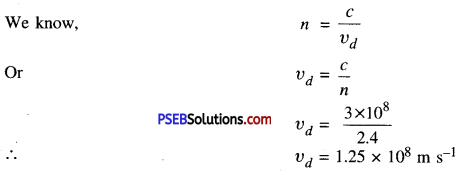Question 6.
Light travels from air to water of refractive index 1.33. Calculate the speed of light in water, if speed of light in air is 3.0 × 108 m/s.
Given : Speed of light in air (c) = 3 × 108 m/s
Refractive index of water aµw – 1.33
Speed of light in water (v) =?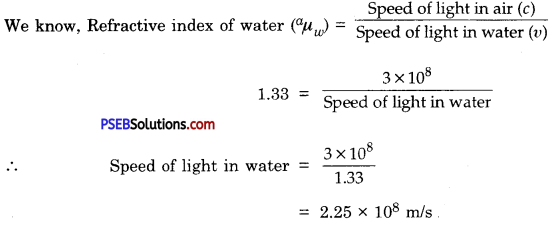Question 7.
Refractive index of water w.r.t. air is $$\frac{4}{3}$$ and that of glass w.r.t air is $$\frac{3}{2}$$ What will be the refractive index of glass w.r.t water?
Here, Refractive Index of water w.r.t. air (aµw) = $$\frac{4}{3}$$
Refractive index of glass w.r.t air (aµg) = $$\frac{3}{2}$$
Refractive index of glass w.r.t water (wµg) =?
Refractive index of glass w.r.t water (wµg) = wµa x aµg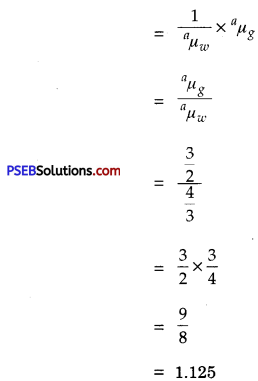Question 8.
An object is 2m away from a lens, which forms an erect image $$\frac{1}{4}$$th the size of the object. Determine the focal length of the lens. What type of the lens is this?
Here, object distance (u) = – 2m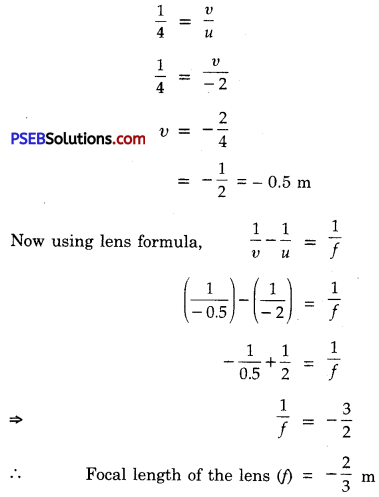Question 9.
A convex lens of focal length 20 cm is placed at a distance of 24 cm from the screen. How far from the lens should an object be placed so as to form a real image on the screen. Also find the nature and magnification of the image produced.
Given : Focal length of the lens (f) = 20 cm
Image distance (v) = 24 cm
Object distance (u) =?
Magnification (m) =?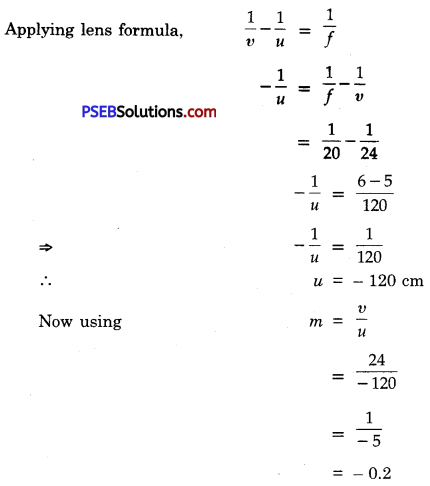Negative sign shows that the image formed is real and inverted.

Question 10.
A concave lens has focal length of 15 cm. At what distance should the object from the lens be placed so that it forms an image at 10 cm from the lens? Also find the magnification produced by the lens.
Given : Focal length of concave lens (f) = – 15 cm
Image distance (v) = – 10 cm
(∵ The image formed in a concave lens is virtual and erect and is formed on the same side of the lens, so it has negative (-) sign.)
Object distance (u) = ?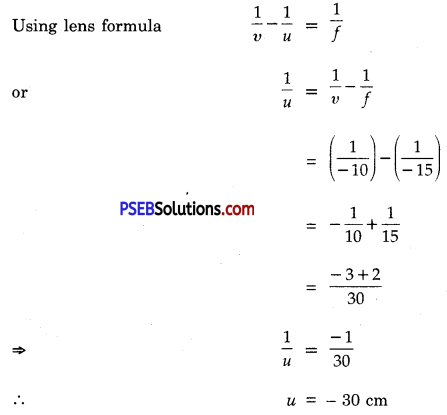Negative sign suggest that the object should be placed at a distance of 30 cm to the left of the lens.
Now magnification (m) = $$-\frac{v}{u}$$
= $$-\frac{(-10 \mathrm{~cm})}{(-30 \mathrm{~cm})}$$
= $$\frac{1}{3}$$
= – 0.33
Negative sign shows that the image is virtual and erect and its size is $$\frac{1}{3}$$rd the size of the object.Question 11.
A convex lens of power 4 D is placed at a distance of 40 cm from a wall. At what distance from the lens should a candle be placed so that its image is formed on the wall?
Given : Power of convex lens(P) = + 4 D
We know P = $$\frac{100}{f(in cm)}$$
f = $$\frac{100}{P}$$
= $$\frac{100}{4}$$ = 25 cm
Image distance (v) = 40cm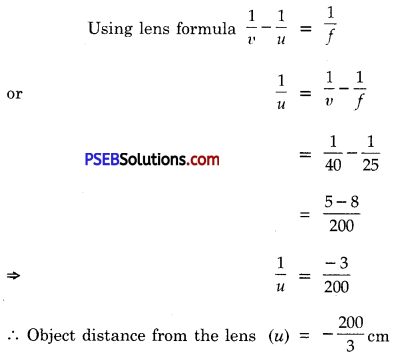Question 1.
Which mirror has a widest field of view?
Convex mirror.

Question 2.
Define a spherical mirror.
Spherical Mirror. A spherical mirror is a part of hollow sphere whose one surface is polished and the other is reflecting.

Question 3.
What is concave mirror?
Concave Mirror. Concave mirror is one which is silvered from outer side and reflection takes place at inner surface.

Question 4.
What is convex mirror?
Convex Mirror. Convex mirror is one which is silvered from inner side and reflection takes place from outer convex side.

Question 5.
Define the pole of a mirror.
Pole. Pole is the mid-point of mirror.

Question 6.
Define principal focus of a mirror.
Principal focus. It is a point on the principal axis at which the ray of light incident in a direction parallel to its axis actually meet or converge or appear to diverge after reflection from the mirror.

Question 7.
Define the focal length of mirror.
Focal Length. It is the distance between pole and principal focus of the mirror.

Question 8.
What is light?
Light. It is a form of energy which causes in us sensation of sight.

Question 9.
What is a real image?
Image formed on a screen is called real image.

Question 10.
What is refraction of light?
The phenomenon of change of path of light from one transparent medium to another is called refraction of light.

Question 11.
Define refractive index?
It is the ratio of sine of the angle of incidence to the sine of the angle of refraction.

Question 12.
What is a lens?
Lens. It is a portion of a transparent medium which is bounded by two surfaces.

Question 13.
Define power of a lens.
Power of lens. It is the ability of a lens to bend the rays.

Question 14.
Define a ‘dioptre’.
Dioptre. It is the power of a lens whose focal length is 1 m.Question 15.
Define focal length of a lens.
Focal length of Lens. The distance between optical centre and principal focus is called focal length.

Question 16.
What is the nature of the mirror having focal length – 15 cm?
It is a concave mirror haying focal length – 15 cm and radius of curvature – 30 cm.

Question 17.
A mirror has magnification 0.4, what type of the mirror is and what type of the image is formed?
It is a convex mirror since the magnification is positive as well as less than one. Image is diminished and erect.

Question 18.
What is curvature of a mirror? What is its value for plane mirror?
Curvature is reciprocal of focal length.
Radius of curvature of a plane mirror is infinity and hence its curvature is = $$\frac{1}{\infty}$$ = zero.

Question 19.
A ray strikes the mirror normally, what is the angle of incidence?
Zero degree. Angle of incidence is the angle which the incident ray makes with the normal.

Multiple Choice Questions :

Question 1.
The power of a lens is 2 Dioptre. Its focal length will be ________

(A) 20cm
(B)4Ocm
(C) 10cm
(D)5Ocm.
(D) 50 cm

Question 2.
________ form.s virtual and same size of image of an object.
(A) Concave mirror
(B) Convex mirror
(C) Plane mirror
(D) None of these.
(C) Plane mirror

Question 3.
The image of an object formed by a convex mirror is always ______
(A) Real, inverted and smaller than the object
(B) Virtual, inverted and smaller than the object
(C) Virtual, erect and smaller than the object
(D) Virtual, erect and bigger than the object.
(C) Virtual, erect and smaller than the object

Question 4.
________ is used in motor vehicles to take rearview.
(A) Concave mirror
(B) Plane mirror
(C) Convex mirror
(D) Any spherical mirror
(C) Convex mirror

Question 5.
$$\frac{\sin i}{\sin r}$$ relation was given by __________
(A) Newton
(B) Raman
(C) Snell
(C) Snell

Question 6.
The focal length of a lens is expressed by which of the following:
(A) $$\frac{1}{f}=\frac{1}{u}+\frac{1}{v}$$
(B) $$\frac{1}{f}=\frac{1}{v}-\frac{1}{u}$$
(C) $$\frac{1}{f}=\frac{1}{v}=\frac{1}{u}$$
(D) $$\frac{1}{f}=\frac{1}{u-frac{1}{v}$$
(B) $$\frac{1}{f}=\frac{1}{v}-\frac{1}{u}$$

Question 7.
The relation between the radius of curvature (R) and focal length (f) of a concave mirror is __________
(A) f = R
(B) f = $$\frac{R}{2}$$
(C) R = $$\frac{f}{2}$$
(D) R = $$\frac{f}{4}$$
(B) f = $$\frac{R}{2}$$

Question 8.
Where will the real and inverted image of an object placed at the centre of curvature of a concave mirror will be formed?
(A) At F
(B) At C
(C) Between C and F
(D) At infinity
(B) At C

Question 9.
The mirror used by dentist is ________
(A) Convex mirror
(B) Concave mirror
(C) Plane mirror
(D) None of the above.
(B) Concave mirrorQuestion 10.
The mirror used for getting real and enlarged image is ________
(A) Convex mirror
(B) Concave mirror
(C) Plane mirror
(D) None of these.
(B) Concave mirror

Question 11.
Parallel rays incident on a mirror, after reflection converge at a point, then the mirror will be _________
(A) Plane
(B) Concave
(C) Convex
(D) None of these.
(B) Concave

Question 12.
The Unit of power of lens is ____________
(A) Coulomb
(B) Watt
(C) Joule
(D) Dioptre
(D) Dioptre

Question 13.
Power of a lens is – 5D, its focal length is :
(A) 20 cm
(B) – 20 cm
(C) – 0.2 m
(D) 5 cm.
(C) – 0.2 m

Question 14.
Which of the following lenses would you prefer to use while reading small letters found in dictionary?
(A) A convex lens of focal length 50 cm
(B) A concave lens of focal length 50 cm
(C) A convex lens of focal length 5 cm
(D) A concave lens of focal length 5 cm
(C)A convex lens of focal length 5 cm

Question 15.
Shaving mirrors are ________
(A) Convex mirrors
(B) Plane mirrors
(C) Concave mirrors
(D) Parabolic mirrors.
(C) Concave mirrors

Question 16.
Which of the following is the property of light?
(A) Reflection
(B) Refraction
(C) Rectilinear propagation
(D) All of these.
(D) All of these.

Question 17.
Twinkling of stars is due to atmospheric :
(A) Reflection of light
(B) Dispersion of light
(C) Interference of light
(D) Refraction of light.
(D) Refraction of light.

Question 18.
Defect of vision that cannot be corrected by spectacles is :
(A) Myopia
(B) Presbyopia
(C) Cataract
(D) Hypermetropia.
(C) Cataract

Question 19.
Which one of the following material cannot be used to make a lens?
(A) Clay
(B) Glass
(C) Water
(D) Plastic.
(A) Clay

Question 20.
Where should object be placed in front of a convex lens to get real image of the size of object?
(A) At principal focus of lens
(B) At twice the focal length
(C) At infinity
(D) Between optical centre of lens and its principal focus. –
(B) At twice the focal length

Question 21.
A spherical mirror and a thin spherical lens have each a focal length -15 cm. The mirror and lens are likely to be _____
(A) Both are convex
(B) Both are concave
(C) Mirror is concave but lens is convex
(D) Mirror is convex and lens is concave.
(B) Both are concave

Question 22.
No matter how far you stand from a mirror, your image appear erect. The mirror is likely to be:
(A) Plane
(B) Concave
(C) Concavo-convex
(D) Convex.
(A) Plane

Question 23.
The refractive index of diamond is:
(A) 2.42
(B) 2.43
(C) 2.45
(D) 2.40.
(A) 2.42

Question 24.
A ray of light travelling in a glass merges into air, it will bend :
(A) Towards the normal
(B) Away from the normal
(C) Goes along the normal
(D) All of these.
(B) Away from the normal.

Fill in the blanks :

Question 1.
A concave mirror forms image inverted and equal in size, when object is placed at ________
centre of curvature (c).

Question 2.
A ________ mirror is used to see the rear view in cars.
convex mirror.Question 3.
The ratio of sine of angle of incidence to the sine of angle of refraction is called ________
Refractive Index.

Question 4.
When a beam of light passes from optically rarer medium to optically denser medium, it bends ________ the normal.